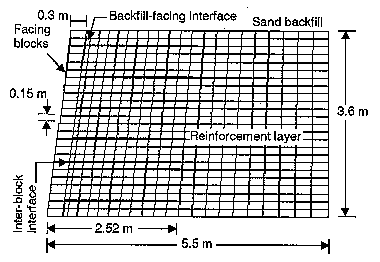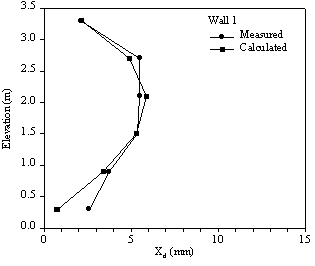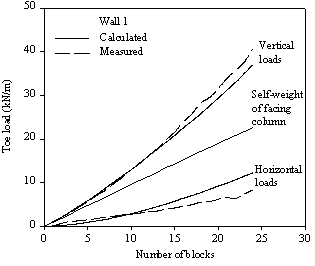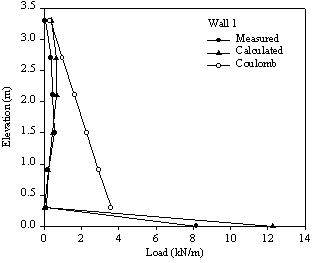# Reinforced soil retaining walls

By Kianoosh Hatami, PhD, PEng

A numerical model has been developed to replicate the measured response of reinforced soil test walls using program FLAC (Itasca 1998).Figure 1: Numerical model of the full scale segmental test wall:

The test walls are a series of carefully instrumented full scale, reinforced soil retaining walls that have been constructed and surcharge loaded by the Geotechnical Research Group at the Royal Military College of Canada (RMC). Primary variables between the test walls include reinforcement type, number of reinforcement layers and facing type. The long term objective of the experimental tests and numerical modeling is to advance the current understanding of reinforced soil wall mechanical behaviour and to develop simple methodologies for analysis and design of reinforced soil walls under service and ultimate loading conditions.

In the following, a sample comparison between the measured and numerically predicted response of one full-scale reinforced soil RMC test wall is presented. The wall under consideration is Wall 1 (the Control Wall) which includes six layers of polypropylene reinforcement at 0.6m vertical spacing.

The dynamic finite difference computer code FLAC (Itasca 1998) was used to simulate the results of the hard-faced walls in this test series. The numerical models used material properties for the backfill soil, reinforcement and modular block interfaces that were obtained from independent laboratory tests.Figure 2: Measured and calculated (numerical) facing block lateral movement at the end of construction for Wall 1:

## Measured and Numerical Results

### Facing Column Profiles and Displacement

Figure 2 shows the profiles of measured and numerically calculated lateral displacements of individual facing blocks at reinforcement elevations for Wall 1 at the end of construction. The measured displacement results are readings from the potentiometers that were positioned against the facing blocks at reinforcement layer levels during construction. Accordingly, the recorded displacement values at each elevation in Figure 3 (image not shown) represent the magnitude of the lateral displacement of the corresponding facing block from the time of installation to the end of construction of Wall 1. The results of facing lateral displacements in Figure 3 (image not shown) show goodFigure 4: Measured and calculated (numerical) horizontal and vertical toe loads for Wall 1 during construction.

### Horizontal and vertical toe loads

Figure 3 (image not shown) shows the history of the measured and calculated (numerical) horizontal and vertical toe loads for Wall 1 during construction. The calculated history of vertical toe reaction during construction shows reasonably good agreement with the measured load data. The predicted history of horizontal toe load is slightly less accurate but captures the trend in the measured data. Superimposed on the figure is the vertical selfweight of the facing column calculated by summing the weights of each individual facing unit. The sum of the measured vertical toe loads is greater than the self-weight of the facing column. This observation is attributed to the vertical down-drag forces developed at the connections due to relative downward movement of the sand backfill directly behind the facing column. This downward movement is a result of compaction of the soil and settlement of the soil during outward rotation of the facing column. While, not shown here, the largest strains in the reinforcement layers at the end of construction were typically located at the connections which is consistent with the down-drag mechanism just described. These additional strains (loads) make it difficult to predict the magnitude and distribution of reinforcement loads in conventional design practice.

### Connection loads

Figure 4 shows measured and calculated (numerical) reinforcement connection loads at the end of construction for Wall 1. The horizontal toe loads are also plotted on the figure to highlight the contribution of toe restraint to stiff facing column reactions. The data indicates that the numerical simulations were capable of capturing both the measured trend and magnitudeFigure 5: Measured, calculated (numerical) and predicted (analytical) reinforcement connection and toe loads at end of construction for Wall 1.

Also plotted on Figure 5 are the predicted connection loads at the end of construction using Coulomb lateral earth pressure theory calculated using the contributory area approach, peak plane strain friction angle of the soil and, the assumption of fully mobilised soil wall friction angle (i.e. ?ps = ? = 44o) (NCMA 1997). In contrast to the expected triangular distribution, the measured connection loads are significantly smaller than the Coulomb predictions and more uniform with depth. The magnitude and distribution of measured connection loads in the hard-faced wall is the combined result of the rigid toe attracting a significant portion of the lateral earth forces and possible redistribution of reinforcement load during construction induced outward movement of the facing column. Similar observations were made for the other hard-faced walls in the test program. Thus, one shortcoming of conventional earth pressure theories applied to reinforced walls with a structural facing is their inability to account for the load that is carried by the restrained toe at the base of a stiff facing column and hence is one source of conservatism in current design practice (i.e. overestimation of reinforcement loads).

## References

1. Itasca Consulting Group 1998. "FLAC - Fast Lagrangian Analysis of Continua", Version 3.40, Itasca Consulting Group, Inc., Minneapolis, Minnesota, USA.
Date modified: